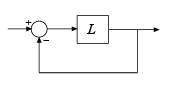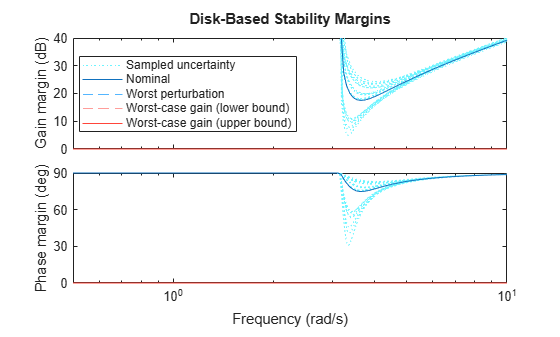# wcdiskmarginplot

Visualize worst-case disk-based stability margins

Since R2020a

## Syntax

``wcdiskmarginplot(Lunc)``
``wcdiskmarginplot(Lunc,sigma)``
``wcdiskmarginplot(___,w)``
``wcdiskmarginplot(___,opts)``

## Description

example

````wcdiskmarginplot(Lunc)` plots the nominal and worst-case disk-based gain and phase margins for the SISO or MIMO negative feedback loop `feedback(Lunc,eye(N))`, where `N` is the number of inputs and outputs in the uncertain open-loop response `Lunc`.For MIMO responses, `diskmarginplot` uses multiloop disk margins. (For details about disk-based gain and phase margins, see `diskmargin`.) The plot includes:Nominal — Nominal gain and phase margins of `Lunc`. The disk-based gain margin at each frequency is ±GM, where GM is the value shown in the plot in dB. Similarly, the disk-based phase margin is ±PM degrees, where PM is the value shown on the plot. Worst perturbation — The disk-based gain and phase margins for the worst perturbation within the uncertainty range `Lunc.Uncertainty`. The worst perturbation corresponds to the `wcu` output argument of `wcdiskmargin`. It is the perturbation that yields the smallest disk margin.Worst-case margin (lower bound) — Lower bound on the worst-case margins at each frequency. This curve represents the envelope produced by finding the smallest disk margin possible at each frequency, within the uncertainty of `Lunc`.Worst-case margin (upper bound) — Upper bound on the worst-case margins at each frequency. Sampled Uncertainty — Margins of responses randomly sampled from `Lunc`.```
````wcdiskmarginplot(Lunc,sigma)` plots the disk-based gain and phase margins computed using the skew `sigma` to bias the gain variation toward gain increase (`sigma` > 0) or gain decrease (`sigma` < 0). If you have used `wc` to obtain worst-case disk-based margins with some particular `sigma`, you can use this syntax to see the frequency dependence of the margins at that `sigma` value. For `sigma` ≠ 0, the plotted value is ```GM = min(gmax,1/max(0,gmin))```. In other words, the plot shows the largest amount of gain change `[1/GM,GM]` that fits within the disk-based gain margin `[gmin,gmax]` of the system at the specified `sigma`. ```

example

````wcdiskmarginplot(___,w)` plots the worst-case margins at the frequencies specified by `w`.If `w` is a cell array of the form `{wmin,wmax}`, then the plot shows the margins at frequencies ranging between `wmin` and `wmax`.If `w` is a vector of frequencies, then the plot shows the margins at each specified frequency.```

example

````wcdiskmarginplot(___,opts)` uses specified options to customize plot elements, aspects of the worst-case margin computation, or both. Use `diskmarginoptions` to specify customizations for the plot. Use `wcOptions` to specify customizations for the computation. You can use this argument with any of the previous syntaxes.```

## Examples

collapse all

Plot the worst-case disk-based gain and phase margins of the following system:

${\mathit{L}}_{\mathrm{unc}}=\frac{1}{{\mathit{s}}^{2}+\mathit{a}+10}\left(1+0.1\Delta \right)$,

where a is an uncertain real parameter with a nominal value of 1 and a range of 0.2–2, and Δ is a gain-bounded dynamic uncertainty.

```a = ureal('a',1,'Range',[.2 2]); Delta = ultidyn('Delta',1); Lunc = tf(1,[1 a 10]) * (1+0.1*Delta); wcdiskmarginplot(Lunc) legend('location','SouthEast')```The `Worst perturbation` curve corresponds to the combination of uncertain elements that yields the smallest disk margin across frequency. This perturbation corresponds to the `wcu` output of `wcdiskmargin`.

The `Worst-case margin` curves show the lower and upper bounds on the worst-case margins at each frequency. For any perturbation within the specified uncertainty range, the disk-based gain or phase margins of the perturbed system lie below the `Worst-case margin` (upper bound) curve. In other words, this curve is the envelope produced by finding the smallest margins within the uncertainty at each frequency. For this system, the lower and upper bounds are close enough to appear identical on the plot. (See `wcdiskmargin` for more information about these bounds.)

Focus the plot on the region between 0.5 and 10 rad/s.

```w = {0.5,10}; wcdiskmarginplot(Lunc,w)```Examine the effect on the worst-case margins of increasing the uncertainty range. To do this without changing the uncertainty specified in `Lunc`, use the `ULevel` option of `wcOptions`. This option scales the normalized uncertainty by the factor you specify. For example, examine the worst-case margins for a 50% greater uncertainty range.

```opts = wcOptions('ULevel',1.5); wcdiskmarginplot(Lunc,w,opts)```In this case, the gain and phase margins for the worst perturbation reach zero, and the worst margin at any frequency is also zero. This result means that extending the uncertainty range this far encompasses some perturbations that drive the closed-loop system `feedback(Lunc,1)` unstable.

Plot the worst-case disk margins as a function of frequency of a system with the following open-loop response.

```a = ureal('a',10,'PlusMinus',[-4,4]); L = tf(25,[1 a a a]);```

For the plot, use the default preferences specified in your Control System Toolbox preference, except specify the following attributes:

• Frequency units: Hz

• Gain margins on a log scale, in absolute units

• Grid on

```opts = diskmarginoptions('cstprefs'); opts.FreqUnits = 'Hz'; opts.MagScale = 'log'; opts.MagUnits = 'abs'; opts.grid = 'on'; w = {2*pi*1e-3,2*pi*10}; % rad/s wcdiskmarginplot(L,w,opts)```The plot you obtain might differ in appearance, depending on your current Control System Toolbox preference settings. (See Toolbox Preferences Editor.)

## Input Arguments

collapse all

Open-loop response, specified as an uncertain model such as a `uss`, `ufrd`, `genss`, or `genfrd` model. `L` can be SISO or MIMO, as long as it has the same number of inputs and outputs. `wcdiskmarginplot` plots the worst-case disk-based gain and phase margins for the negative-feedback closed-loop system `feedback(L,eye(N))`.To plot the worst-case margins of the positive feedback system `feedback(L,eye(N),+1)`, use `wcdiskmargin(-L)`.

If `L` is a frequency-response data model (such as `ufrd`), then `wcdiskmarginplot` plots the margins at each frequency represented in the model.

Skew of uncertainty region used to compute the stability margins, specified as a real scalar. This parameter biases the uncertainty used to model gain and phase variations toward gain increase or gain decrease.

• The default `sigma` = 0 uses a balanced model of gain variation in a range `[gmin,gmax]`, with ```gmin = 1/gmax```.

• Positive `sigma` uses a model with more gain increase than decrease (`gmin > 1/gmax`).

• Negative `sigma` uses a model with more gain decrease than increase (`gmin < 1/gmax`).

For more detailed information about how the choice of `sigma` affects the margin computation, see Stability Analysis Using Disk Margins.

When plotting the gain margins of a dynamic system versus frequency, use the default `sigma` = 0 to get unbiased estimates of gain and phase margins. For `sigma` = 0, the worst-case disk-based gain margin at each frequency is ±GM, where GM is the value shown in the plot in dB.

If you have used `wcdiskmargin` to obtain worst-case disk-based margins with some particular `sigma`, you can use this syntax to see the frequency dependence of the margins at that `sigma` value. For `sigma` ≠ 0, plotted value is ```GM = min(gmax,1/max(0,gmin))```. In other words, the plot shows the largest amount of gain change `[1/GM,GM]` that fits within the disk-based gain margin `[gmin,gmax]` of the system at the specified sigma.

Frequencies at which to plot stability margins, specified as the cell array `{wmin,wmax}` or as a vector of frequency values.

• If `w` is a cell array of the form `{wmin,wmax}`, then the plot shows the margins at frequencies between `wmin` and `wmax`.

• If `w` is a vector of frequencies, then the plot shows the margins at each specified frequency. For example, use `logspace` to generate a row vector with logarithmically spaced frequency values.

Specify frequencies in units of rad/`TimeUnit`, where `TimeUnit` is the `TimeUnit` property of `L`.

Plot options, specified as:

• A `diskmarginplot` options set that you create with `diskmarginoptions`. Use these options to customize aspects of plot appearance such as title, axis labels, and grids.

• A `wcOptions` options set. Use these options to customize aspects of the worst-case margins computation, such as scaling the uncertainty to examine the effect of smaller or larger uncertainty range without changing the uncertainty levels in `Lunc`.

• One of each type of options set, to specify both plot options and computation options. Separate the two options sets by a comma, as in `wcdiskmarginplot(Lunc,w,plotops,compopts)`.

## Version History

Introduced in R2020a